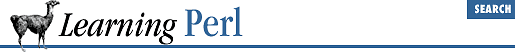home | O'Reilly's CD bookshelfs | FreeBSD | Linux | Cisco | Cisco Exam

### 8.5 Private Variables in Functions

We've already talked about the ``` @_``` variable and how a local copy gets created for each subroutine invoked with parameters. You can create your own scalar, array, and hash variables that work the same way. You do this with the ``` my``` operator, which takes a list of variable names and creates local versions of them (or instantiations , if you like bigger words). Here's that ``` add``` function again, this time using ``` my``` :

```sub add {
my (\$sum);           # make \$sum a local variable
\$sum = 0;            # initialize the sum
foreach \$_ (@_) {
\$sum += \$_;      # add each element
}
return \$sum;            # last expression evaluated: sum of all elements
}```

When the first body statement is executed, any current value of the global variable ``` \$sum``` is saved away, and a brand new variable named ``` \$sum``` is created (with the value ``` undef``` ). When the subroutine exits, Perl discards the local variable and restores the previous (global) value. This works even if the ``` \$sum``` variable is currently a local variable from another subroutine (a subroutine that invokes this one, or one that invokes one that invokes this one, and so on). Variables can have many nested local versions, although you can access only one at a time.

Here's a way to create a list of all the elements of an array greater than 100:

```sub bigger_than_100 {
my (@result);             # temporary for holding the return value
foreach \$_ (@_) {         # step through the arg list
if (\$_ > 100) {       # is it eligible?
push(@result,\$_); # add it
}
}
return @result;           # return the final list
}```

What if we wanted all elements greater than 50 rather than greater than 100? We'd have to edit the program, changing the 100's to 50's. But what if we needed both? Well, we can replace the 50 or 100 with a variable reference instead. This makes it look like:

```sub bigger_than {
my(\$n,@values);           # create some local variables
(\$n,@values) = @_;        # split args into limit and values
my(@result);              # temporary for holding the return value
foreach \$_ (@values) {    # step through the arg list
if (\$_ > \$n) {        # is it eligible?
push(@result,\$_); # add it
}
}
return @result;                  # return the final list
}
# some invocations:
@new = bigger_than(100,@list);    # @new gets all @list > 100
@this = bigger_than(5,1,5,15,30); # @this gets (15,30)```

Notice that this time we used two additional local variables to give names to arguments. This is fairly common in practice; it's much easier to talk about ``` \$n``` and ``` @values``` than to talk about ``` \$_``` and ``` @_[1..\$#]``` , and safer, too.

The result of ``` my``` is an assignable list, meaning that it can be used on the left side of an array assignment operator. This list can be given initial values for each of the newly created variables. (If you don't give values to the list, the new variables start with a value of ``` undef``` , just like any other new variable.) This means we can combine the first two statements of this subroutine, replacing:

```my(\$n,@values);
(\$n,@values) = @_; # split args into limit and values```

with:

`my(\$n,@values)= @_;`

This is, in fact, a very common Perl-ish thing to do. Local nonargument variables can be given literal values in the same way, such as:

`my(\$sum) = 0; # initialize local variable`

Be warned that despite its appearances as a declaration, ``` my``` is really an executable operator. Good Perl hacking strategy suggests that you bunch all your ``` my``` operators at the beginning of the subroutine definition, before you get into the meat of the routine.8.4 Arguments8.6 Semiprivate Variables Using local